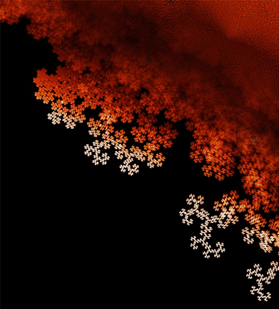# Simple Math Expressions Yield Intricate Visual Patterns

John Matson in Scientific American:On December 5 John Baez, a mathematical physicist at the University of California, Riverside, posted a collection of images of polynomial roots by Dan Christensen, a mathematician at the University of Western Ontario, and Sam Derbyshire, an undergraduate student at the University of Warwick in England.

Polynomials are mathematical expressions that in their prototypical form can be described by the sum or product of one or more variables raised to various powers. As a single-variable example, take x2 – x – 2. This expression is a second-degree polynomial, or a quadratic, meaning that the variable (x) is raised to the second power in the term with the largest exponent (x2).

A root of such a polynomial is a value for x such that the expression is equal to zero. In the quadratic above, the roots are 2 and –1. That is to say, plug either of those numbers in for x and the polynomial will be equal to zero. (These roots can be found by using the famous quadratic formula.) But some roots are more complex. Take the quadratic polynomial x2 + 1. Such an expression is only equal to zero when x2 is equal to –1, but on its face this seems impossible. After all, a positive number times a positive number is positive, and a negative number times a negative number is positive as well. So what number, multiplied by itself, could be negative?

More here, including slide show.